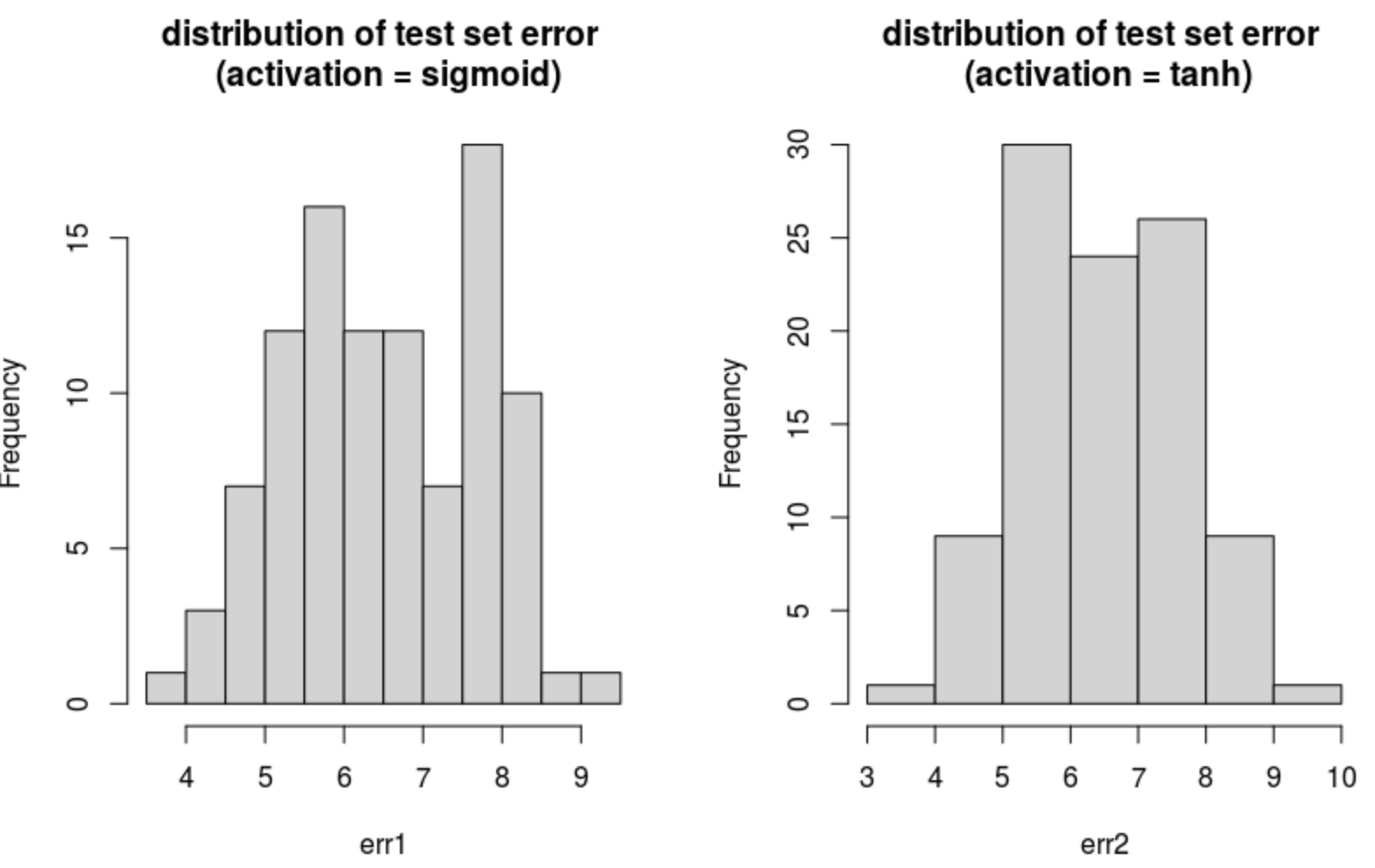In previous posts, I introduced LSBoost; a gradient boosting machine that uses randomized and penalized least squares as a basis – instead of decision trees which are frequently used as base learners. mlsauce’s LSBoost takes into account a problem’s nonlinearity by including new, engineered explanatory variables $$g(XW+b)$$ with:

• $$g$$: an activation function (tanh, ReLU, sigmoid, …)
• $$X$$: input data (covariates, explanatory variables)
• $$W$$: a matrix containing numbers drawn from a multivariate uniform distribution on $$[0, 1]$$

New activation functions were added to version 0.8.0 of mlsauce: ReLU6, tanh, sigmoid. These changes are available both in R and in the Python implementation of mlsauce.

The following R example illustrates the differences between out-of-sample errors, when $$g$$ = sigmoid or $$g$$ = tanh. Of course, LSBoost can be tuned further than what’s demonstrated here.

library(devtools)
devtools::install_github("Techtonique/mlsauce/R-package")
library(mlsauce)

# Input data
X <- as.matrix(MASS::Boston[, -1])
y <- as.integer(MASS::Boston[, 1])

n <- dim(X)
p <- dim(X)

# number of repeats for obtaining the distribution of errors
n_repeats <- 100

# function for calculating the out-of-sample error, based on activation functions
get_rmse_error <- function(activation = c("sigmoid", "tanh", "relu6", "relu"))
{
err <- rep(0, n_repeats)

pb <- txtProgressBar(min = 0, max = n_repeats, style = 3)
for (i in 1:n_repeats)
{
set.seed(21341+i*10)
train_index <- sample(x = 1:n, size = floor(0.8*n), replace = TRUE)
test_index <- -train_index
X_train <- as.matrix(X[train_index, ])
y_train <- as.double(y[train_index])
X_test <- as.matrix(X[test_index, ])
y_test <- as.double(y[test_index])

# using default parameters
obj <- mlsauce::LSBoostRegressor(verbose = FALSE,
activation = match.arg(activation))

obj$fit(X_train, y_train) err[i] <- sqrt(mean((obj$predict(X_test) - y_test)**2))

setTxtProgressBar(pb, i)
}

return(err)

}

# test set error for g=sigmoid
(err1 <- get_rmse_error("sigmoid"))
# test set error for g=tanh
(err2 <- get_rmse_error("tanh"))

# distribution of test set error
par(mfrow=c(1, 2))
hist(err1, main = "distribution of test set error \n (activation = sigmoid)")
hist(err2, main = "distribution of test set error \n (activation = tanh)")> print(sessionInfo())
R version 4.0.3 (2020-10-10)
Platform: x86_64-pc-linux-gnu (64-bit)
Running under: Ubuntu 16.04.7 LTS

Matrix products: default
BLAS:   /usr/lib/atlas-base/atlas/libblas.so.3.0
LAPACK: /usr/lib/atlas-base/atlas/liblapack.so.3.0

locale:
 LC_CTYPE=C.UTF-8       LC_NUMERIC=C           LC_TIME=C.UTF-8
 LC_COLLATE=C.UTF-8     LC_MONETARY=C.UTF-8    LC_MESSAGES=C.UTF-8